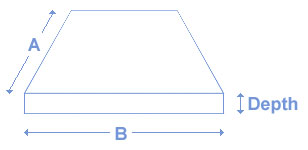### Total Cubic Yards

To use this cubic yard calculator, enter the width (in feet), length (in feet), and depth (in inches) of the area you would like filled. Hit the “Calculate” button. The Calculated Cubic Yards of Each material will be displayed to the right.### Calculated Total Cubic Yards

*These calculations are to be used a guide, they do not guarantee exact totals necessary.

### Calculated Total Shape

*These calculations are to be used a guide, they do not guarantee exact totals necessary.

### Circle Calculator

To use this calculator, enter the diameter (in feet), and thickness (in inches) of the area you would like filled. Hit the “Calculate” button. The tonnage of Each material will be displayed to the left.